Distortion Characteristics of Paralleled Tubes

There have been reports that paralleling small signal tubes doesn't sound as good as a single section by itself. This page provides data on a 12AX7 (NOS Sylvania) configured as a conventional cathode biased amplifier. Five graphs of distortion by component (2nd thru 9th) are presented for the following conditions:

1. Conventional Amplifier. Cathode Bypassed, plate resistor 100k, cathode bias resistor 1.8k.

2. Same as above, except both sections are placed in parallel.

3. Same as (2), plate resistor changed to 50k, cathode resistor to 900 ohms. This ought to be similar in performance to (1), but it isn't.

4. Same as (3), but the cathode is unbypassed.

5. Same as (1), but the cathode is unbypassed.

In all cases, the plate supply voltage was 250 volts, and the data plots distortion form 5 volts (RMS) to 40 volts.

Figure 1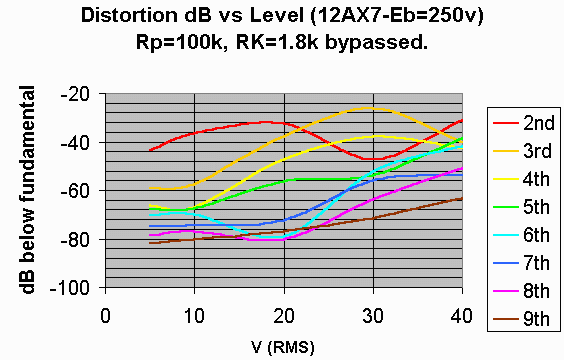Figure 2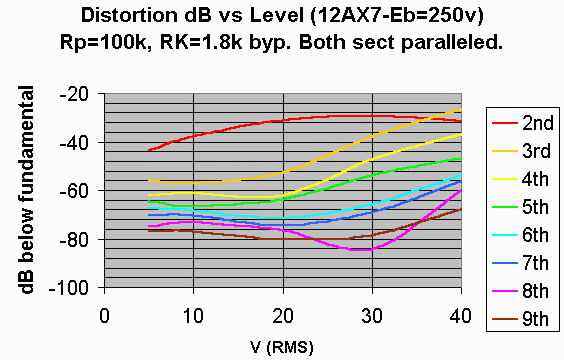Figure 3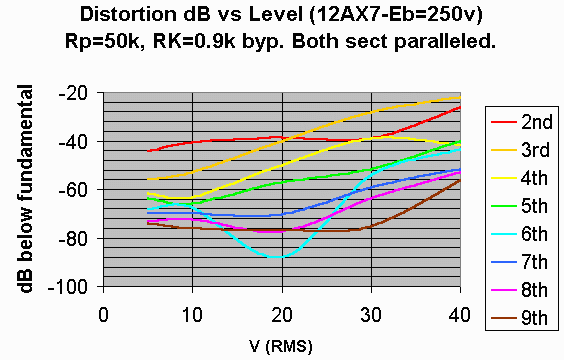Figure 4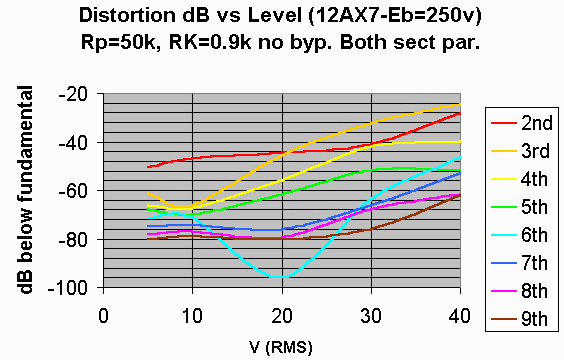Figure 5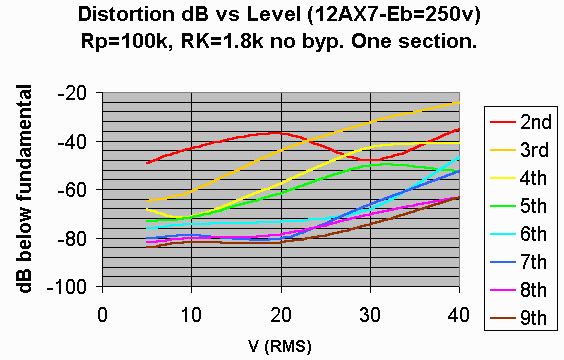Discussion

At "normal" signal levels, paralleling both sections of the tube tends to increase the higher order distortion. This can be seen by comparing figures 1 and 2. But in some sense, this is an unfair comparison, since each section of the tube is now operating at half the current of the single tube. To rectify this, compare figures 1 and 3. Now something even worse shows up, the high order distortion products are increased at the expense of the second  (which is reduced). This may not be of very good benefit.

If it is necessary to parallel sections (for instance, to reduce noise in low level sections), keeping at least some portion of the cathodes unbypassed reduces the effect. Compare, for instance, figure 4 to figure 5.

There is also another benefit in the case of paralleled sections. There is no case where the distortion increases when the bypass capacitor is removed  (compare figure 3 to figure 4). This is NOT true of the standard single section amplifier (compare figure 1 to figure 5). Note that at 30 volts, the 5th harmonic is substantially more prominent with the bypass capacitor removed.

Conclusions:

Paralleling sections of tubes may not sound as good as a single section by itself. However, it seems as though this is one case where unbypassed cathode operation is sonically justified.